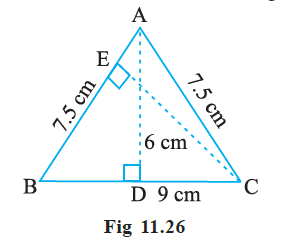# ΔABC is isosceles with AB = AC = 7.5 cm and BC = 9 cm (Figure). The height AD from A to BC, is 6 cm. Find the area of ΔABC. What will be the height from C to AB i.e., CE?Solution

From the given figure we note that

AB = AC = 7.5 cm

BC = 9 cm

We know that

Area of ΔABC = ½ × base × height

= ½ × BC × AD

= ½ × 9 × 6

= 1 × 9 × 3

= 27 cm2

Also

Area of ΔABC = ½ × base × height

27 = ½ × AB × CE

27 = ½ × 7.5 × CE

(27 × 2)/7.5 = CE

CE = 54/7.5

CE = 7.2 cm(0)(0)